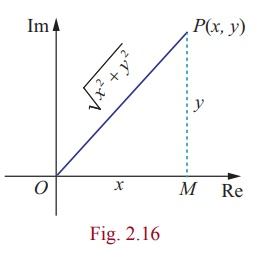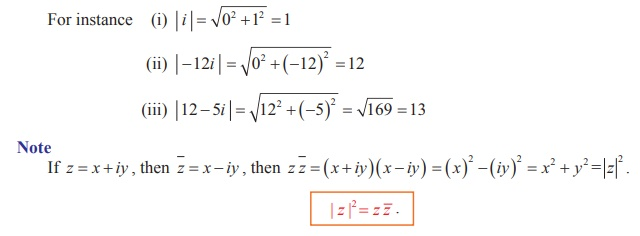Home | | Maths 12th Std | Modulus of a Complex Number

# Modulus of a Complex Number

If z = x + iy, then the modulus of z, denoted by |z| , is defined by |z|

Modulus of a Complex Number

Just as the absolute value of a real number measures the distance of that number from origin along the real number line, the modulus of a complex number measures the distance of that number from the origin in the complex plane. Observe that the length of the line from the origin along the radial line to z = x + iy is simply the hypotenuse of a right triangle, with one side of length x and the other side of lengthDefinition 2.4

If z = x + iy, then the modulus of z, denoted by |z| , is defined by |z| = ŌłÜ[x2 + y2]Tags : Definition, Formulas , 12th Mathematics : UNIT 2 : Complex Numbers
Study Material, Lecturing Notes, Assignment, Reference, Wiki description explanation, brief detail
12th Mathematics : UNIT 2 : Complex Numbers : Modulus of a Complex Number | Definition, Formulas